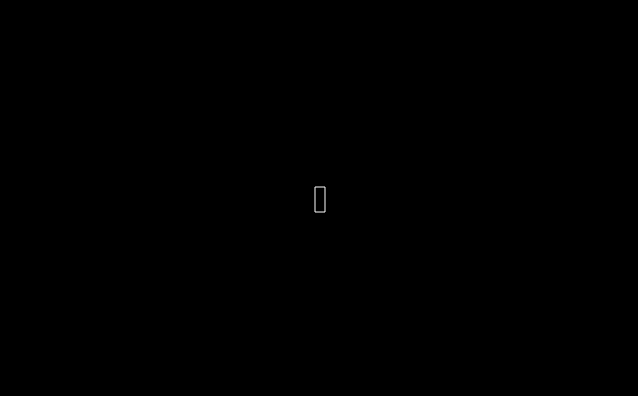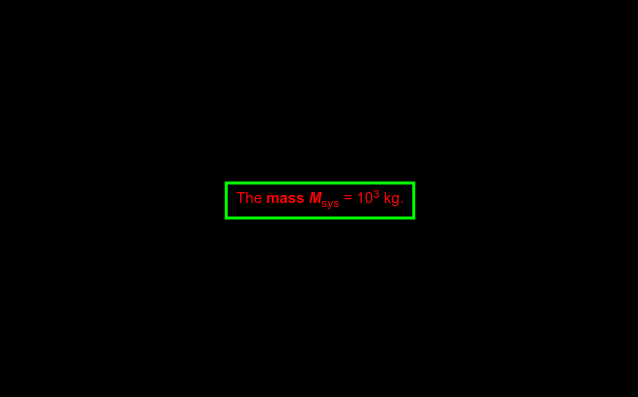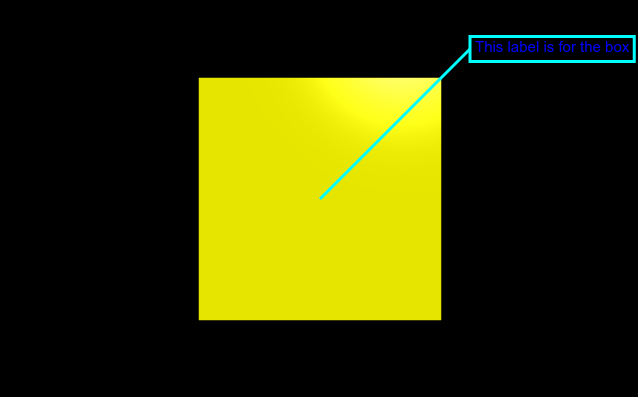# Making a label with VPython

`VPython` makes it easy to create navigable 3D displays and animations, even for those with limited programming experience. Because it is based on Python, it also has much to offer for experienced programmers and researchers. `VPython` allows users to create objects such as spheres and cones in 3D space and displays these objects in a window. This makes it easy to create simple visualizations, allowing programmers to focus more on the computational aspect of their programs. The simplicity of `VPython` has made it a tool for the illustration of simple physics, especially in the educational environment.

Installation :

`pip install vpython`

A label object is used to display text in a box. The label will always face forward even if the canvas is rotated. We can generate a label in `VPython` using the `label()` method.

## label() method

Syntax : cylinder(parameters)

Parameters :

• pos : It is the point in the world space being labelled. Assign a vector containing 3 values, example pos = vector(0, 0, 0) or the object being labeled, example pos = obj.pos
• pixel_pos : It determines the position in terms of pixels. Assign a boolean value
• align : It is the alignment of the label. Assign a string with either of the options, “center”, “right” and “left”, default is “center”
• color : It is the color of the text of the label. Assign a vector containing 3 values, example color = vector(1, 1, 1) will give the color white
• background : It is the color of the background of the label. Assign a vector containing 3 values, example color = vector(1, 1, 1) will give the background color white
• opacity : It is the opacity of the background of the box. Assign a floating value in which 1 is the most opaque and 0 the least opaque, example opacity = 0.5
• xoffset : It is the offset for the the x axis of the label. Assign a floating value, example xoffset = 2
• yoffset : It is the offset for the the y axis of the label. Assign a floating value, example yoffset = 5
• text : It is the text to be displayed in the label. HTML styles can also be included while assigning the text.
• font : It is the font of the text of the label. Assign a string value, the default is “sans”, example font = “serif”
• height : It is the height of the font in pixels. Assign a integer value, the default is 15, example height = 18
• border : It is the distance in pixels from the text to the surrounding box. Assign a floating value, the default length is 5, example border = 10
• radius : It is the radius of the cylinder. Assign a floating value, the default radius is 1, example radius = 5
• box : It determines whether the box should be drawn or not. Assign a boolean value in which True is yes and False is no, the default is True
• line : It determines whether a line from the pos to the box should be drawn or not. Assign a boolean value in which True is yes and False is no, the default is True
• linecolor : It is the color of the line and the box. Assign a vector containing 3 values, example color = vector(1, 1, 1) will give the linecolor white
• linewidth : It is the thickness of the line drawn from the pos to the box, and the edges of the box. Assign a integer value, the default is 1 pixel, example linewidth = 5
• space : It is the radius in pixels of a sphere surrounding pos, into which the connecting line does not go. Assign a integer value, example space = 20
• visible : It determines whether the label is to displayed or not. Assign a boolean value in which True is yes and False is no, the default is True

All the parameters are optional.

Example 1 :A label with no parameters, all the parameters will have the default value.

 `# import the module ` `from` `vpython ``import` `*` `label() `

Output :Example 2 :A label using the parameters color, text, linewidth, linecolor and border.

 `# import the module ` `from` `vpython ``import` `*` `label(text ``=` `"The mass Msys = 103 kg."``, ` `      ``color ``=` `vector(``1``, ``0``, ``0``), ` `      ``linecolor ``=` `vector(``0``, ``1``, ``0``), ` `      ``linewidth ``=` `3``, ` `      ``border ``=` `10``)  `

Output :Example 3 :A label for an object.

 `# import the module ` `from` `vpython ``import` `*` ` `  `# the box to be labelled ` `b ``=` `box(color ``=` `vector(``1``, ``1``, ``0``), ` `        ``size ``=` `vector(``1``, ``1``, ``1``)) ` ` `  `# the label for the box ` `label(pos ``=` `b.pos, ` `      ``text ``=` `"This label is for the box"``, ` `      ``font ``=` `"sans"``, ` `      ``color ``=` `vector(``0``, ``0``, ``1``), ` `      ``linecolor ``=` `vector(``0``, ``1``, ``1``), ` `      ``linewidth ``=` `3``, ` `      ``yoffset ``=` `150``, ` `      ``xoffset ``=` `150``)  `

Output :My Personal Notes arrow_drop_upCheck out this Author's contributed articles.

If you like GeeksforGeeks and would like to contribute, you can also write an article using contribute.geeksforgeeks.org or mail your article to contribute@geeksforgeeks.org. See your article appearing on the GeeksforGeeks main page and help other Geeks.

Please Improve this article if you find anything incorrect by clicking on the "Improve Article" button below.

Article Tags :

Be the First to upvote.

Please write to us at contribute@geeksforgeeks.org to report any issue with the above content.